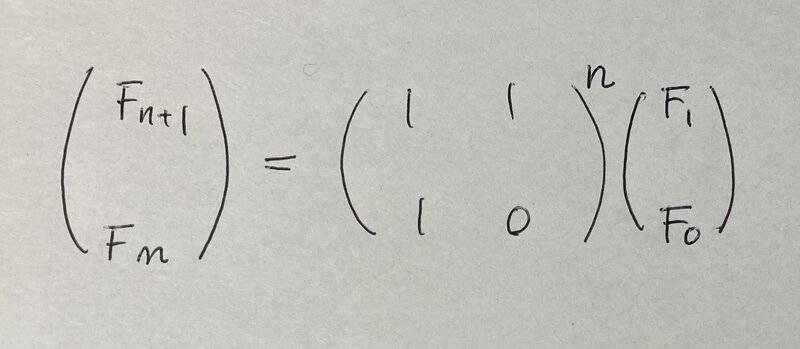# Matrix representation for closed-form expression for Fibonacci numbers

• I
• murshid_islam

#### murshid_islam

TL;DR Summary
Matrix representation for closed-form expression for Fibonacci numbers:
From the wikipedia page for Fibonacci numbers, I got that the matrix representation for closed-form expression for Fibonacci numbers is:

$$\begin{pmatrix} 1 & 1 \\ 1 & 0\\ \end{pmatrix} ^ n = \begin{pmatrix} F_{n+1} & F_n \\ F_n & F_{n-1}\\ \end{pmatrix}$$

That only works when $F_0 = 0$ and $F_1 = 1$. How can I find the matrix representation for arbitrary starting values, for example, when $F_0 = a$ and $F_1 = b$?

Summary:: Matrix representation for closed-form expression for Fibonacci numbers:

From the wikipedia page for Fibonacci numbers, I got that the matrix representation for closed-form expression for Fibonacci numbers is:

$$\begin{pmatrix} 1 & 1 \\ 1 & 0\\ \end{pmatrix} ^ n = \begin{pmatrix} F_{n+1} & F_n \\ F_n & F_{n-1}\\ \end{pmatrix}$$

That only works when $F_0 = 0$ and $F_1 = 1$. How can I find the matrix representation for arbitrary starting values, for example, when $F_0 = a$ and $F_1 = b$?
$$\begin{pmatrix} a+b & b \\ b & a\\ \end{pmatrix} = \begin{pmatrix} F_2 & F_1 \\ F_1 & F_0\\ \end{pmatrix}$$

Then multiply repeatedly on the left by
$$\begin{pmatrix} 1 & 1 \\ 1 & 0\\ \end{pmatrix}$$

•PeroK and murshid_islam
Though same as post #2,•martinbn and murshid_islam